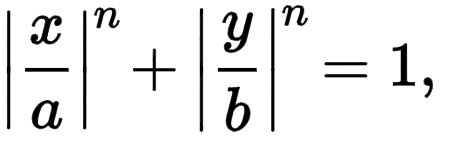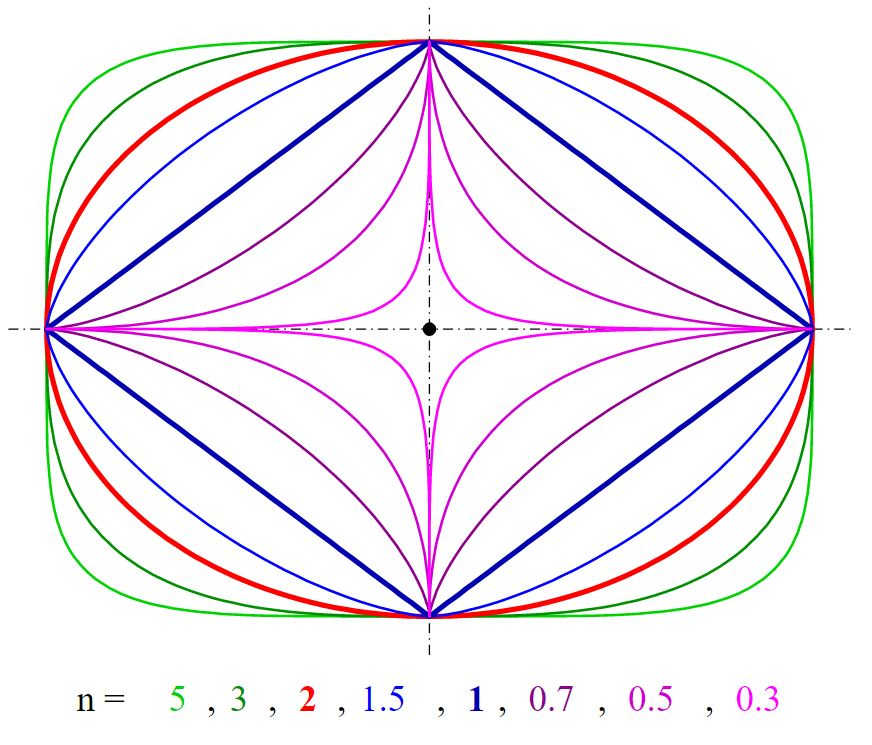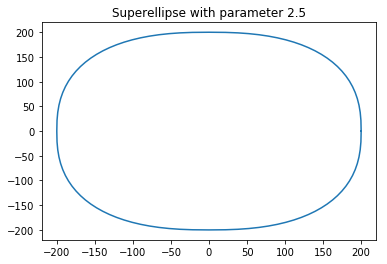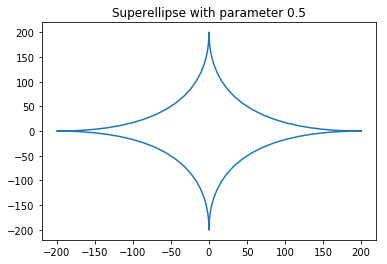# Implementing a Superellipse

What is a superellipse
A superellipse, also known as a Lamé curve after Gabriel Lamé, is a closed curve resembling the ellipse, retaining the geometric features of semi-major axis and semi-minor axis, and symmetry about them, but a different overall shape.
In the Cartesian coordinate system, the set of all points (x, y) on the curve satisfy the equationwhere n, a and b are positive numbers, and the vertical bars | | around a number indicate the absolute value of the number.a = 1 and b = 0.75

There are many number of specific cases of superellipse like given in the image down below:These can be achieved by varying the value of n in the equation. So now we try to implement this in python and to do that we are require some libraries.

Modules Required:

• matplotlib: To plot the curve of the equation. Its an 3rd party library in python and if you want to install it click here.
• math : Its an built in library of python which have almost all the mathematical tools.

 `# Python program to implement  ` `# Superellipse ` ` `  `# importing the required libraries ` `import` `matplotlib.pyplot as plt ` `from` `math ``import` `sin, cos, pi ` `  `  `def` `sgn(x): ` `    ``return` `((x>``0``)``-``(x<``0``))``*``1` ` `  `# parameter for marking the shape   ` `a, b, n ``=` `200``, ``200``, ``2.5` `na ``=` `2` `/` `n ` `# defining the accuracy ` `step ``=` `100`  `piece ``=``(pi ``*` `2``)``/``step ` `xp ``=``[];yp ``=``[] ` `  `  `t ``=` `0` `for` `t1 ``in` `range``(step ``+` `1``): ` `    ``# because sin ^ n(x) is mathematically the same as (sin(x))^n... ` `    ``x ``=``(``abs``((cos(t)))``*``*``na)``*``a ``*` `sgn(cos(t)) ` `    ``y ``=``(``abs``((sin(t)))``*``*``na)``*``b ``*` `sgn(sin(t)) ` `    ``xp.append(x);yp.append(y) ` `    ``t``+``=` `piece ` `  `  `plt.plot(xp, yp) ``# plotting all point from array xp, yp ` `plt.title(``"Superellipse with parameter "``+``str``(n)) ` `plt.show() `

Output:when n = 2.5

Now let’s see what happens when we changes the value of n to 0.5

 `# Python program to implement  ` `# Superellipse ` `# importing the required libraries ` `import` `matplotlib.pyplot as plt ` `from` `math ``import` `sin, cos, pi ` `  `  `def` `sgn(x): ` `    ``return` `((x>``0``)``-``(x<``0``))``*``1` ` `  `# parameter for marking the shape   ` `a, b, n ``=` `200``, ``200``, ``0.5` `na ``=` `2` `/` `n ` `# defining the accuracy ` `step ``=` `100`  `piece ``=``(pi ``*` `2``)``/``step ` `xp ``=``[];yp ``=``[] ` `  `  `t ``=` `0` `for` `t1 ``in` `range``(step ``+` `1``): ` `    ``# because sin ^ n(x) is mathematically the same as (sin(x))^n... ` `    ``x ``=``(``abs``((cos(t)))``*``*``na)``*``a ``*` `sgn(cos(t)) ` `    ``y ``=``(``abs``((sin(t)))``*``*``na)``*``b ``*` `sgn(sin(t)) ` `    ``xp.append(x);yp.append(y) ` `    ``t``+``=` `piece ` `  `  `plt.plot(xp, yp) ``# plotting all point from array xp, yp ` `plt.title(``"Superellipse with parameter "``+``str``(n)) ` `plt.show() `

Output:Source Code of the program in Java.

 `// Java program to implement ` `// Superellipse ` `import` `java.awt.*; ` `import` `java.awt.geom.Path2D; ` `import` `static` `java.lang.Math.pow; ` `import` `java.util.Hashtable; ` `import` `javax.swing.*; ` `import` `javax.swing.event.*; ` ` `  `public` `class` `SuperEllipse ``extends` `JPanel ``implements` `ChangeListener { ` `    ``private` `double` `exp = ``2.5``; ` ` `  `    ``public` `SuperEllipse() ` `    ``{ ` `        ``setPreferredSize(``new` `Dimension(``650``, ``650``)); ` `        ``setBackground(Color.white); ` `        ``setFont(``new` `Font(``"Serif"``, Font.PLAIN, ``18``)); ` `    ``} ` ` `  `    ``void` `drawGrid(Graphics2D g) ` `    ``{ ` `        ``g.setStroke(``new` `BasicStroke(``2``)); ` `        ``g.setColor(``new` `Color(``0xEEEEEE``)); ` ` `  `        ``int` `w = getWidth(); ` `        ``int` `h = getHeight(); ` `        ``int` `spacing = ``25``; ` ` `  `        ``for` `(``int` `i = ``0``; i < w / spacing; i++) { ` `            ``g.drawLine(``0``, i * spacing, w, i * spacing); ` `            ``g.drawLine(i * spacing, ``0``, i * spacing, w); ` `        ``} ` `        ``g.drawLine(``0``, h - ``1``, w, h - ``1``); ` ` `  `        ``g.setColor(``new` `Color(``0xAAAAAA``)); ` `        ``g.drawLine(``0``, w / ``2``, w, w / ``2``); ` `        ``g.drawLine(w / ``2``, ``0``, w / ``2``, w); ` `    ``} ` ` `  `    ``void` `drawLegend(Graphics2D g) ` `    ``{ ` `        ``g.setColor(Color.black); ` `        ``g.setFont(getFont()); ` `        ``g.drawString(``"n = "` `+ String.valueOf(exp), getWidth() - ``150``, ``45``); ` `        ``g.drawString(``"a = b = 200"``, getWidth() - ``150``, ``75``); ` `    ``} ` ` `  `    ``void` `drawEllipse(Graphics2D g) ` `    ``{ ` ` `  `        ``final` `int` `a = ``200``; ``// a = b ` `        ``double``[] points = ``new` `double``[a + ``1``]; ` ` `  `        ``Path2D p = ``new` `Path2D.Double(); ` `        ``p.moveTo(a, ``0``); ` ` `  `        ``// calculate first quadrant ` `        ``for` `(``int` `x = a; x >= ``0``; x--) { ` `            ``points[x] = pow(pow(a, exp) - pow(x, exp), ``1` `/ exp); ``// solve for y ` `            ``p.lineTo(x, -points[x]); ` `        ``} ` ` `  `        ``// mirror to others ` `        ``for` `(``int` `x = ``0``; x <= a; x++) ` `            ``p.lineTo(x, points[x]); ` ` `  `        ``for` `(``int` `x = a; x >= ``0``; x--) ` `            ``p.lineTo(-x, points[x]); ` ` `  `        ``for` `(``int` `x = ``0``; x <= a; x++) ` `            ``p.lineTo(-x, -points[x]); ` ` `  `        ``g.translate(getWidth() / ``2``, getHeight() / ``2``); ` `        ``g.setStroke(``new` `BasicStroke(``2``)); ` ` `  `        ``g.setColor(``new` `Color(``0x25B0C4DE``, ``true``)); ` `        ``g.fill(p); ` ` `  `        ``g.setColor(``new` `Color(``0xB0C4DE``)); ``// LightSteelBlue ` `        ``g.draw(p); ` `    ``} ` ` `  `    ``@Override` `    ``public` `void` `paintComponent(Graphics gg) ` `    ``{ ` `        ``super``.paintComponent(gg); ` `        ``Graphics2D g = (Graphics2D)gg; ` `        ``g.setRenderingHint(RenderingHints.KEY_ANTIALIASING, ` `                           ``RenderingHints.VALUE_ANTIALIAS_ON); ` `        ``g.setRenderingHint(RenderingHints.KEY_TEXT_ANTIALIASING, ` `                           ``RenderingHints.VALUE_TEXT_ANTIALIAS_ON); ` ` `  `        ``drawGrid(g); ` `        ``drawLegend(g); ` `        ``drawEllipse(g); ` `    ``} ` ` `  `    ``@Override` `    ``public` `void` `stateChanged(ChangeEvent e) ` `    ``{ ` `        ``JSlider source = (JSlider)e.getSource(); ` `        ``exp = source.getValue() / ``2.0``; ` `        ``repaint(); ` `    ``} ` ` `  `    ``public` `static` `void` `main(String[] args) ` `    ``{ ` `        ``SwingUtilities.invokeLater(() -> { ` `            ``JFrame f = ``new` `JFrame(); ` `            ``f.setDefaultCloseOperation(JFrame.EXIT_ON_CLOSE); ` `            ``f.setTitle(``"Super Ellipse"``); ` `            ``f.setResizable(``false``); ` `            ``SuperEllipse panel = ``new` `SuperEllipse(); ` `            ``f.add(panel, BorderLayout.CENTER); ` ` `  `            ``JSlider exponent = ``new` `JSlider(JSlider.HORIZONTAL, ``1``, ``9``, ``5``); ` `            ``exponent.addChangeListener(panel); ` `            ``exponent.setMajorTickSpacing(``1``); ` `            ``exponent.setPaintLabels(``true``); ` `            ``exponent.setBackground(Color.white); ` `            ``exponent.setBorder(BorderFactory.createEmptyBorder(``20``, ``20``, ``20``, ``20``)); ` ` `  `            ``Hashtable labelTable = ``new` `Hashtable<>(); ` `            ``for` `(``int` `i = ``1``; i < ``10``; i++) ` `                ``labelTable.put(i, ``new` `JLabel(String.valueOf(i * ``0.5``))); ` `            ``exponent.setLabelTable(labelTable); ` ` `  `            ``f.add(exponent, BorderLayout.SOUTH); ` ` `  `            ``f.pack(); ` `            ``f.setLocationRelativeTo(``null``); ` `            ``f.setVisible(``true``); ` `        ``}); ` `    ``} ` `} `

Output:

This article is contributed by Subhajit Saha. If you like GeeksforGeeks and would like to contribute, you can also write an article using contribute.geeksforgeeks.org or mail your article to contribute@geeksforgeeks.org. See your article appearing on the GeeksforGeeks main page and help other Geeks.

Attention reader! Don’t stop learning now. Get hold of all the important DSA concepts with the DSA Self Paced Course at a student-friendly price and become industry ready.

My Personal Notes arrow_drop_upCheck out this Author's contributed articles.

If you like GeeksforGeeks and would like to contribute, you can also write an article using contribute.geeksforgeeks.org or mail your article to contribute@geeksforgeeks.org. See your article appearing on the GeeksforGeeks main page and help other Geeks.

Please Improve this article if you find anything incorrect by clicking on the "Improve Article" button below.

Article Tags :
Practice Tags :

Be the First to upvote.

Please write to us at contribute@geeksforgeeks.org to report any issue with the above content.